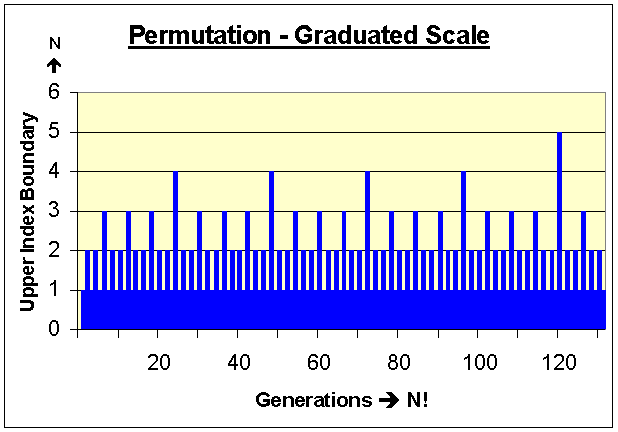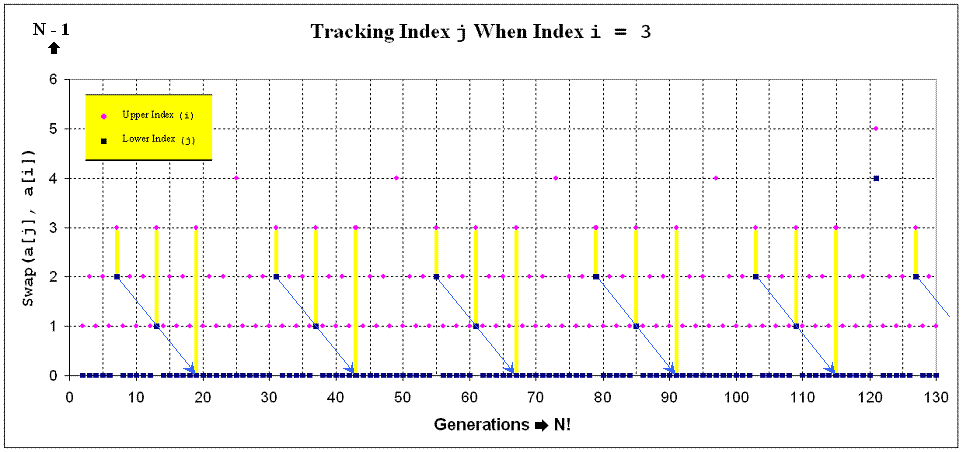# The Countdown QuickPerm Algorithm (Head):(Formally Example_01)

A complete permutation over a very large array is time consuming to say the least. Without a strategic plan, a permutation effort will be fruitless. In the algorithm presented below, exchanges are concentrated within the head of the target array such that one swap produces a new ordered list. Index values will not advance until all combinations are exhausted inside of the established range. Specifically, repeated access to an established upper index peak must be counted. Only when this count equals the value of the upper index in question can the upper index value proceed to the next available index level.

Consider the following example regarding a prioritized list. Imagine that your house keys were lost while surfing the net at a friend's house. Where would you begin your search? Would your search begin

a.) near the computer?
d.) at a neighbor’s house?

Odds are you would retrace your steps by first searching your pockets and the surrounding area repeatedly long before you would even search the neighbor’s house. Here choice "a" is intentionally placed first immediately followed by an ordered list of less likely choices (by degree). Choices very similar to these can be initially prioritized within an array before a permutation is even attempted. Frequently permutations begin with a known solution and attempt to improve upon agreeable ideas as permutable subsets. Simply stated, time management is vital when dealing with very large arrays or large character strings.

Furthermore, assume that a fast computer can complete a permutation set using a 50-element target array in exactly 10 seconds. It's also reasonable to assume this computer can complete a permutation set using a larger target array within a greater time period. Using the presented scenario above and the known factorial properties of a permutation, the following conclusions can be established:

A permutation over a new 51-element array would complete in approximately:

10 Seconds * 51 = 510 Seconds or 8.5 Minutes

A permutation over a new 52-element array would complete in approximately:

10 Seconds * 51 * 52 = 26520 Seconds or 442 Minutes or 7.37 Hours
OR 8.5 Minutes * 52 = 442 Minutes or 7.37 Hours

A permutation over a new 53-element array would complete in approximately:

10 Seconds * 51 * 52 * 53 = 1405560 Seconds or 16.27 Days

A permutation over a new 54-element array would complete in approximately:

10 Seconds * 51 * 52 * 53 * 54 = 75900240 Seconds or 2.41 Years

A permutation over a new 55-element array would complete in approximately:

10 Seconds * 51 * 52 * 53 * 54 * 55 = 4174513200 Seconds or 132.37 Years

In the above scenario, an absolute solution can probably be identified within a 53-element array. Using an array with more than 53-elements would cause an unreasonable and predictable wait. The QuickPerm algorithm presented below uses permutation subsets on the head of the target array. Often the target array a[N] is simply used to index large complex data structures. Here's the actual QuickPerm C++ algorithm for your review:

QuickPerm - Head Permutations Using a Linear Array Without Recursion
 ``````// NOTICE: Copyright 1991-2010, Phillip Paul Fuchs #define N 12 // number of elements to permute. Let N > 2 void QuickPerm(void) { unsigned int a[N], p[N+1]; register unsigned int i, j, tmp; // Upper Index i; Lower Index j for(i = 0; i < N; i++) // initialize arrays; a[N] can be any type { a[i] = i + 1; // a[i] value is not revealed and can be arbitrary p[i] = i; } p[N] = N; // p[N] > 0 controls iteration and the index boundary for i //display(a, 0, 0); // remove comment to display array a[] i = 1; // setup first swap points to be 1 and 0 respectively (i & j) while(i < N) { p[i]--; // decrease index "weight" for i by one j = i % 2 * p[i]; // IF i is odd then j = p[i] otherwise j = 0 tmp = a[j]; // swap(a[j], a[i]) a[j] = a[i]; a[i] = tmp; //display(a, j, i); // remove comment to display target array a[] i = 1; // reset index i to 1 (assumed) while (!p[i]) // while (p[i] == 0) { p[i] = i; // reset p[i] zero value i++; // set new index value for i (increase by one) } // while(!p[i]) } // while(i < N) } // QuickPerm() ``````
QuickPerm - Function to Display Permutations (Optional)
 ``````// NOTICE: Copyright 1991-2010, Phillip Paul Fuchs void display(unsigned int *a, unsigned int j, unsigned int i)             { for(unsigned int x = 0; x < N; x++) printf("%d ",a[x]); printf(" swapped(%d, %d)\n", j, i); getch(); // press any key to continue... } // display() ``````

The primary index controller array in the QuickPerm algorithm above is p[N], which controls iteration and the upper index boundary for variable i. This array is initialized to corresponding index values so that a simple countdown process can be utilized. The controller array is analogous to an incremental base N odometer, where the least significant digit p is always zero, digit (or wheel) p counts in base 2 or binary, digit p counts in base 3, ..., and digit p[N] counts in base N+1. Initial values are assigned as follows:

p = 0
p = 1
p = 2
...
p[N] = N

Initially the default upper index variable i is set to one as a reference to the binary odometer digit which in-turn is immediately reduced by one (a countdown process). Since the upper index variable is clearly odd, the default lower index variable j is assigned to the odometer digit referenced by the upper index which coincides to the value now stored in p[i] or zero. Both index variables are properly set and the first SWAP is called...

Before another swap can take place, upper and lower index values must be recalculated based upon values stored in the base N odometer p[N]. Again the upper index begins at the binary digit p and resets each consecutive ZERO digit to a corresponding index value (maximum digit). The upper index will settle upon the first odometer digit that is not zero then reduce the referenced digit by one (again a true countdown process). If the upper index is even, the lower index is assigned to the least significant odometer digit which coincides to the value stored in p (or simply the lower index will remain at zero). Otherwise the lower index will be assigned to the actual odometer digit now referenced by the upper index. A SWAP is executed and this process continues until the upper index attempts to reference an odometer digit beyond the length of the linear permutable target in question.

Notice the lower index always follows the upper index's lead. Observable modifications compared to the counting QuickPerm algorithm include the following:

1. Initializing all integers in the controller array to corresponding index values.
2. Decrement p[i] by one before the conditional assignment j = p[i]
3. While p[i] equals 0 DO reset p[i] to i then increment i by one.

Using a nested while loop instead of a conditional if-else statement (as described in the counting QuickPerm algorithm) clearly lead to the development of a Meta-permutation class. Although this conditional substitution can be made regardless of the counting process utilized, the Meta-permutation class precludes such a substitution in order to return valid indices.

Fundamental Analysis of QuickPerm Above {Switch to Characters}
 Number of Objectsto Permute (N) a[N] Display ofInitial Sequence a[N] Display ofFinal Sequence Total Number of UniqueCombinations Produced (N!) 3   (Client/HTML) 1 2 3 3 2 1 2 * 3 = 6 4   (Client/HTML) 1 2 3 4 4 1 2 3 6 * 4 = 24 5   (Server/PHP) 1 2 3 4 5 5 2 3 4 1 24 * 5 = 120 6   (Server/PHP) 1 2 3 4 5 6 6 3 4 1 2 5 120 * 6 = 720 7   (Server/PHP) 1 2 3 4 5 6 7 7 2 3 4 5 6 1 720 * 7 = 5040 8   (Server/PHP) 1 2 3 4 5 6 7 8 8 3 4 5 6 1 2 7 5040 * 8 = 40320 9   (Client/JavaScript) 1 2 3 4 5 6 7 8 9 9 2 3 4 5 6 7 8 1 40320 * 9 = 362880 if N is even 1 2 3 . . . (N - 1) N N 3 4 . . . (N - 2) 1 2 (N - 1) (N - 1)! * N = N! if N is odd 1 2 3 . . . (N - 1) N N 2 3 . . . (N - 1) 1 (N - 1)! * N = N!

A Permutation Ruler:The graph below reflects how the value stored in the lower index variable j consistently reduces by one when the upper index variable i is odd, otherwise j is assigned a zero value. For each reference to j when i is odd, the lower index variable j will be assigned to the upper index variable “i – 1” then “i – 2” until the lower index variable j reaches zero. To control this iteration behavior, the value stored in the controller array p[i] is initialized to the same value stored in the upper index variable i (as mentioned above) then repeatedly reduced by one and assigned to the lower index variable j. This process continues until the lower index variable j reaches 0 then repeats. (Please note, the lower index variable j can also begin at zero and advance by one until the upper index value is reached.) This behavior is strictly controlled by the following statement:

IF i is odd then j = p[i] otherwise j = 0

Which in-turn translates to the following C++ statement:

j = i % 2 * p[i];